## AngouriMath with F# for Research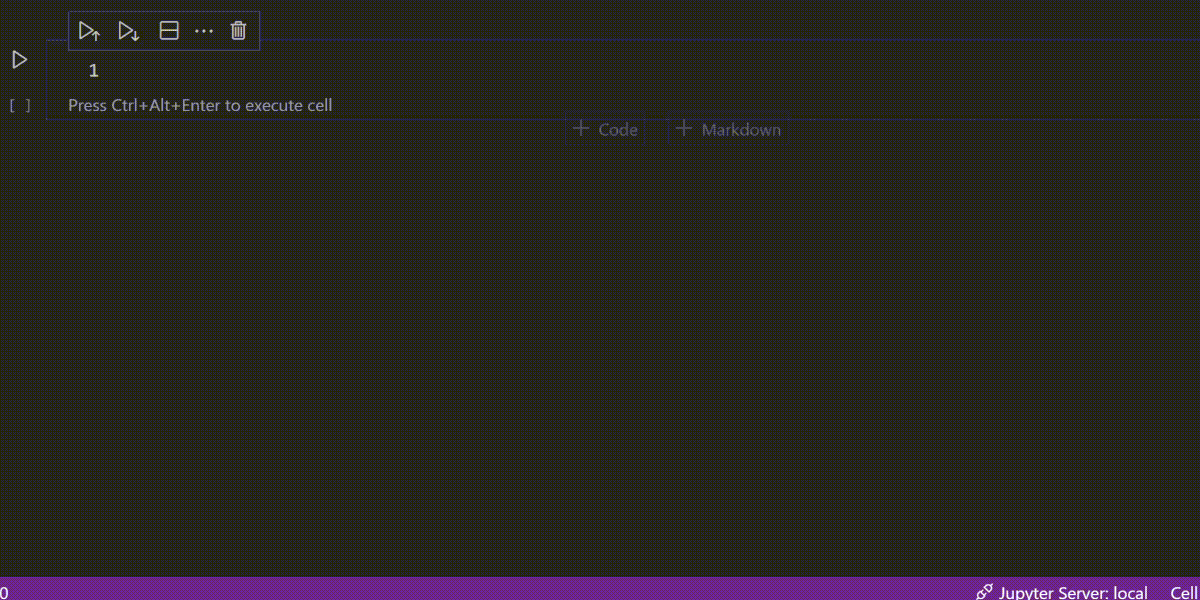AngouriMath.Interactive is suited for interactive computations and research, as it exposes a good amount of F# API. Basic functions include simplification, differentiation, latexisation (getting a LaTeX string from an expression), as well as solving equations, integrations, systems, working with vectors and matrices, etc.

Its main usage is notebooks, which are usable from Jupyter and Visual Studio Code notebooks extension. See dotnet/interactive to get notebooks working for you too.

### Getting started

To get AngouriMath in your notebook, install it by running a so-called magic command:

#r "nuget:AngouriMath.Interactive,*-*"

Now, let's run a hello world:

open AngouriMath.FSharp.Functions
open AngouriMath.FSharp.Core
let x = symbol "x"
let a = symbol "a"
let expr = sin x + a
expr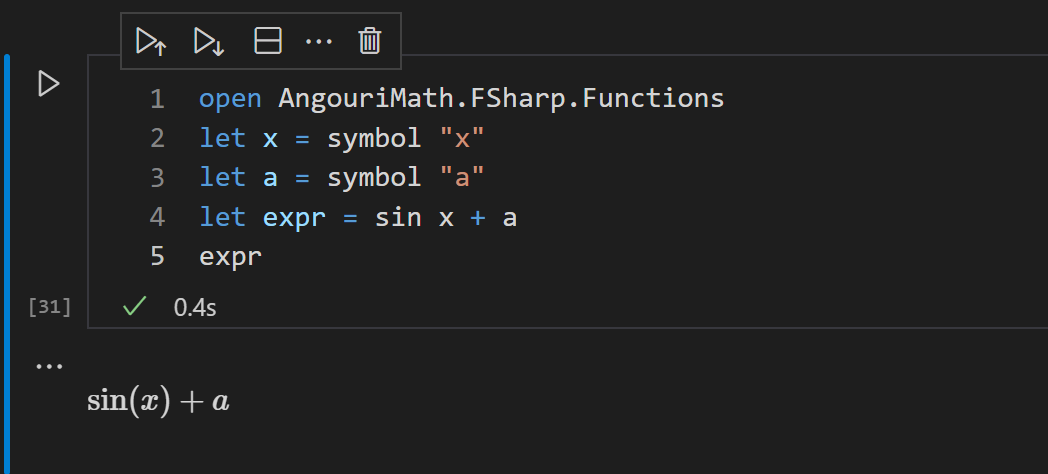Let's find a derivative and integral over x, and their simplified versions:

derivative x expr + integral x expr
derivative x expr + integral x expr |> simplified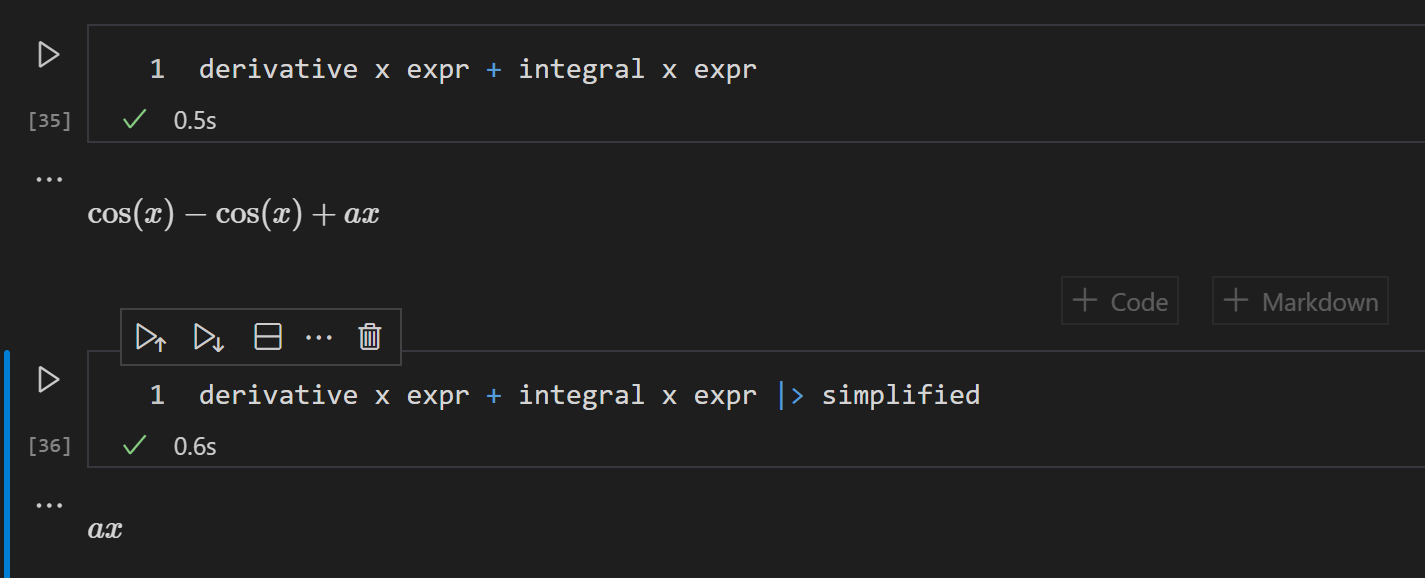Now, let's construct something more complex. For example, an equation:

let Omega_lambda = symbol "Omega_lambda"
let Omega_1 = symbol "Omega_1"
let eq = equality Omega_1 (sin Omega_lambda + sin (parsed 2 * Omega_lambda))
eq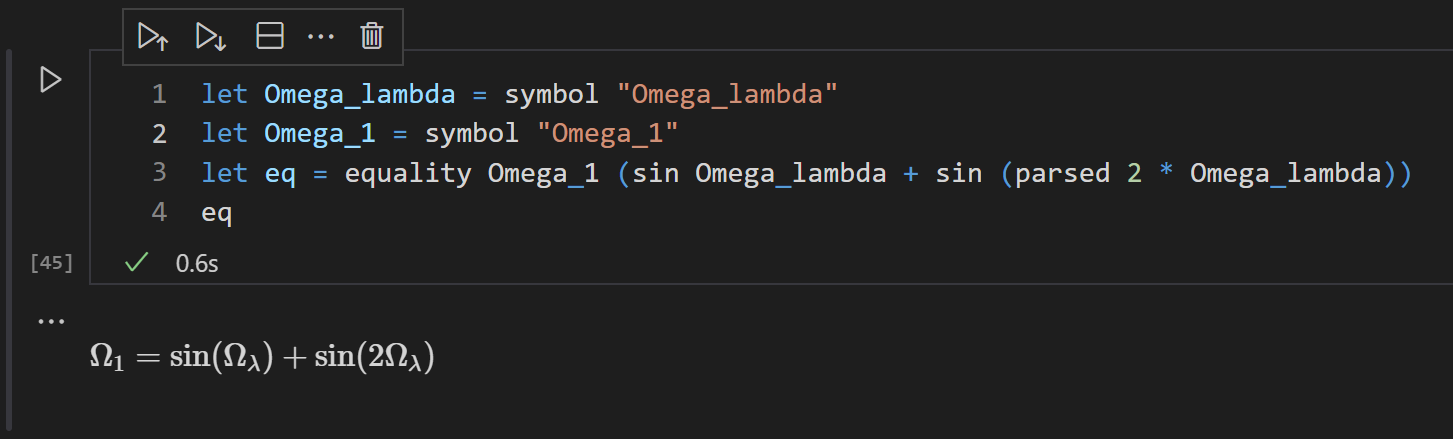Let's find the solutions over Omega_lambda:

let roots = solutions Omega_lambda eq
roots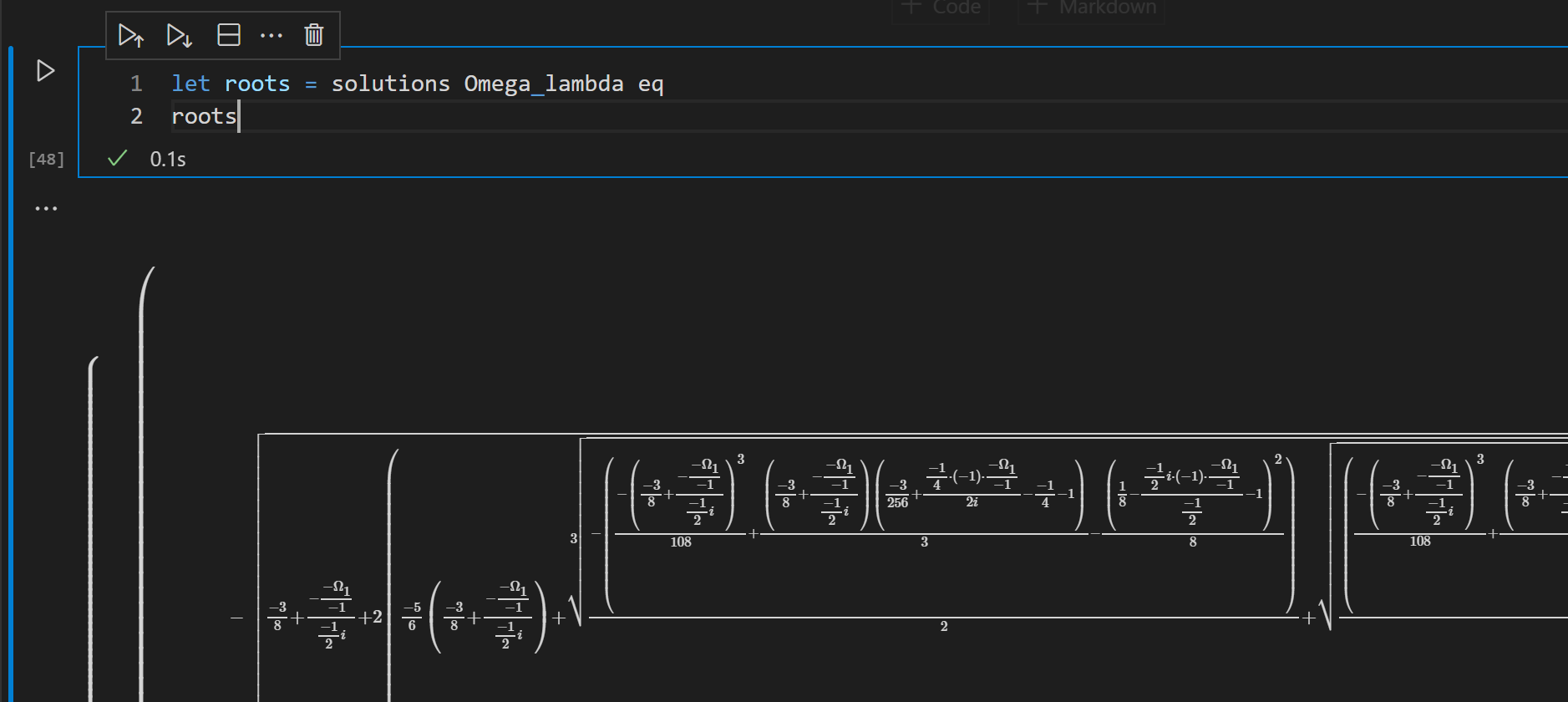This is a set of roots, which is too huge for a screenshot. The only variable is Omega_1, so let's substitute it with a numeric value and evaluate the roots, showing them line by line with the default precision:

open AngouriMath
open AngouriMath.FSharp.Shortcuts
roots <|- (Omega_1, 0.3) |> evaled :?> Entity.Set.FiniteSet
|> Seq.map (fun el -> el.ToString()) |> String.concat "\n"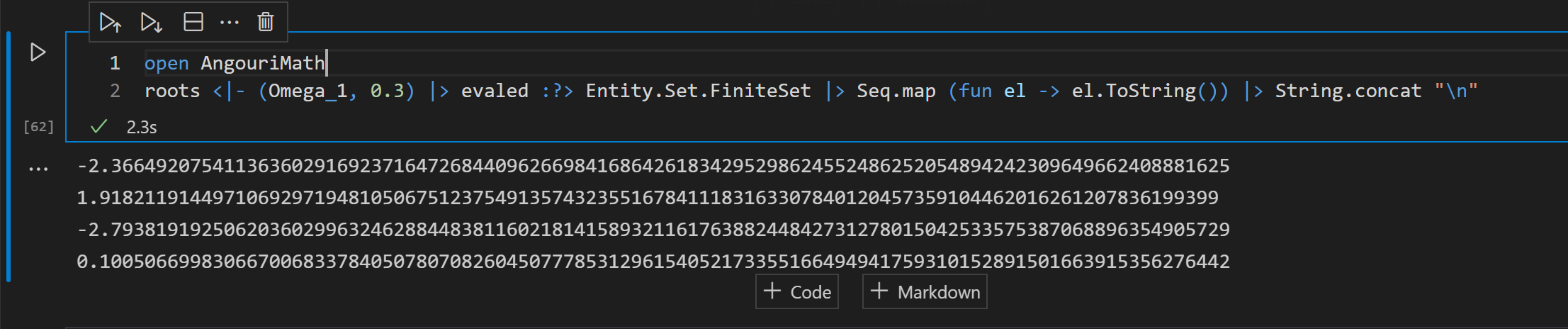### Visualization

With the help of great plotting library Plotly.NET, it is possible to visualize functions over two and three variables.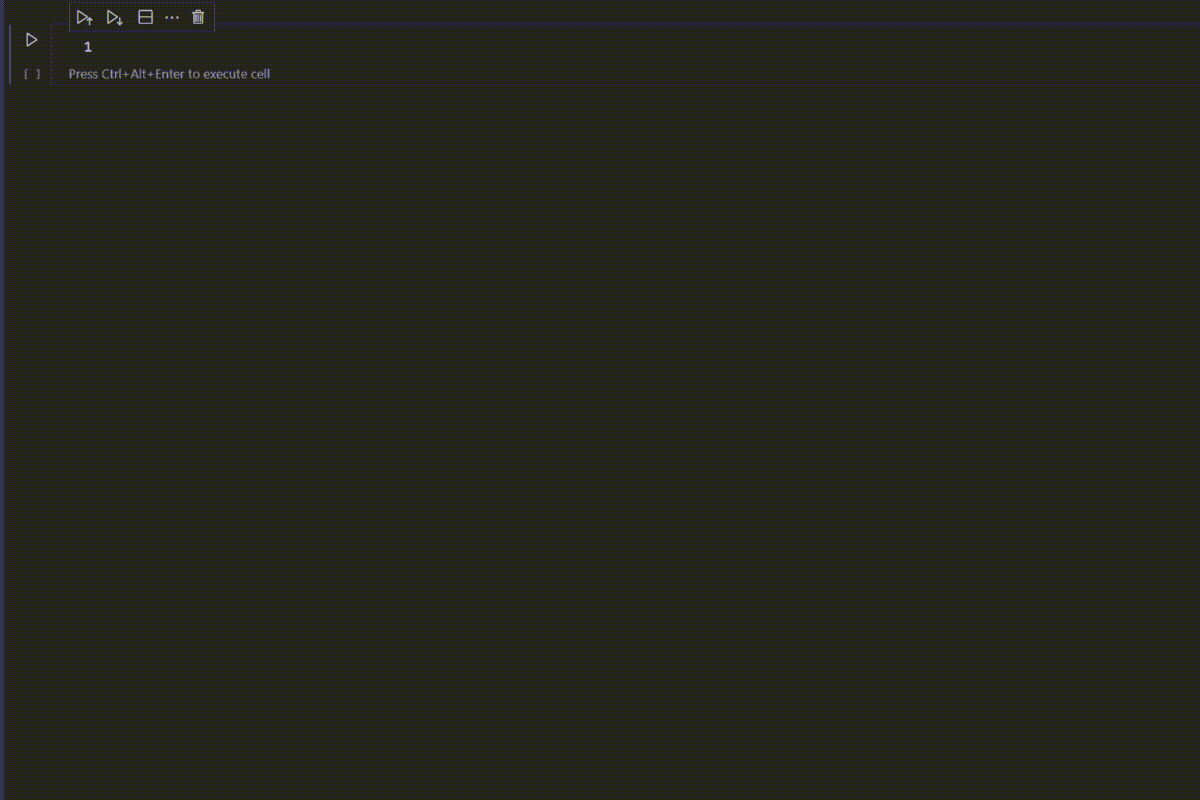Now, let's do some basic plotting. There is a few functions in the AngouriMath.Interactive namespace. Plot.linear and Plot.scatter visualizes single-variable functions:

open AngouriMath.Interactive
Plot.linear [ 0.0..0.01..3. ] "sin(x) + sin(2x) + sin(3x) + sin(4x)"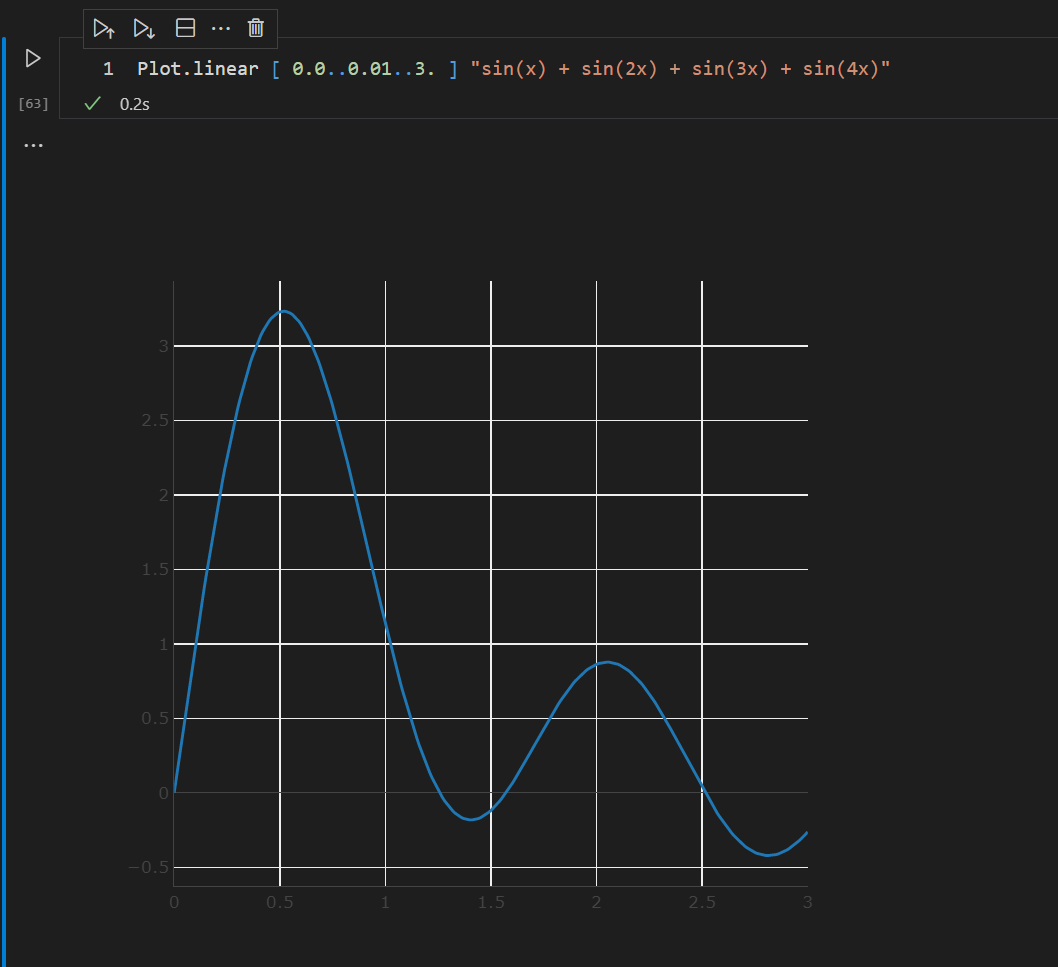Now, let's display a surface, descriped by a function of two variables:

let f1 = parsed "sin(x) + sin(2x) + sin(3x) + sin(4x) + sin(5x)"
let f2 = parsed "cos(y) + sin(2y) + cos(3y) + sin(4y) + cos(5y)"
let z = f1 * f2
Plot.surface [ 0.0..0.01..3.5 ] [ 0.0..0.02..3.5 ] z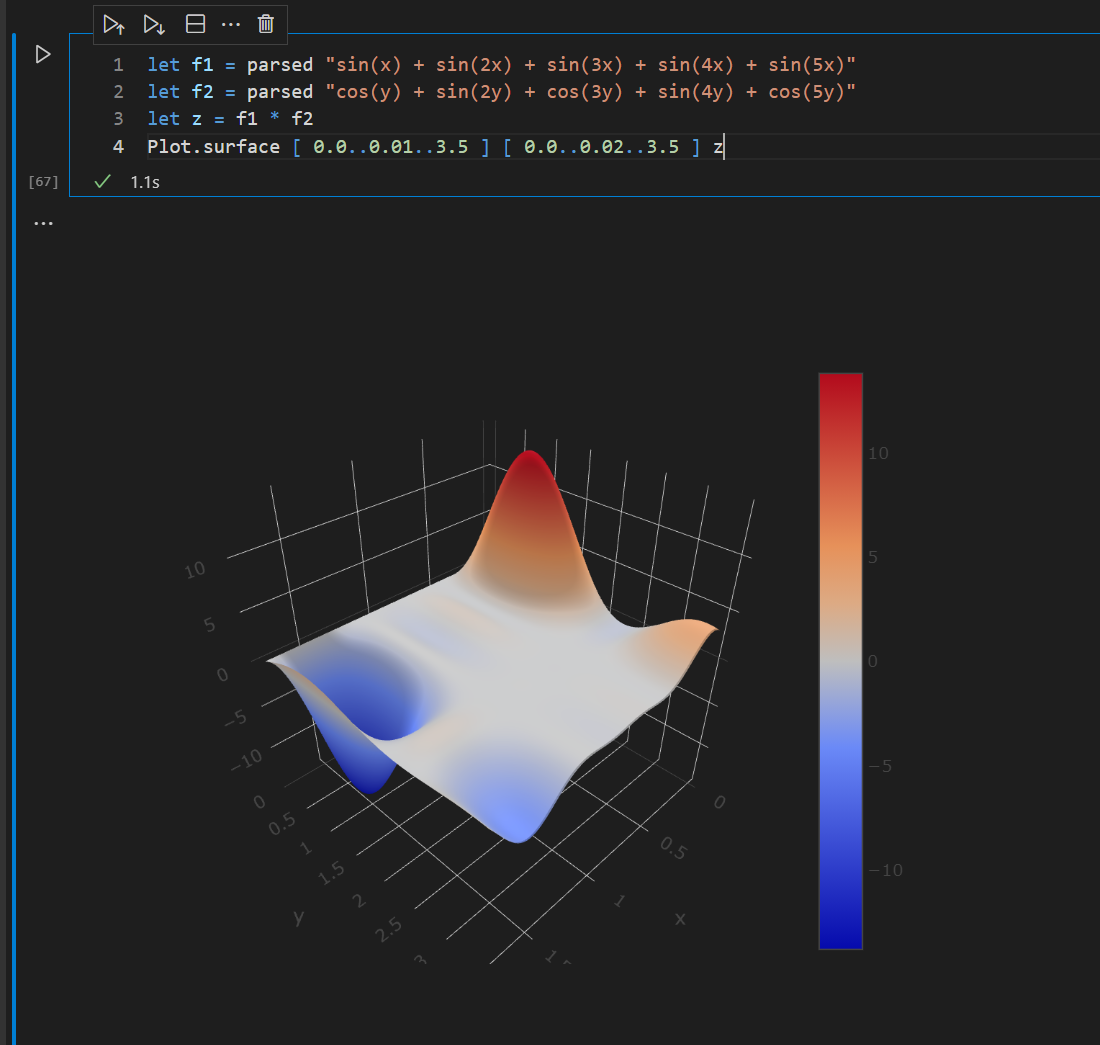Plotting is available since 1.4.0-preview.1

### Terminal

AngouriMath.Terminal is convenient CLI for interacting with functions without running a notebook, creating a file, etc. The commands ran in the terminal are in F# too.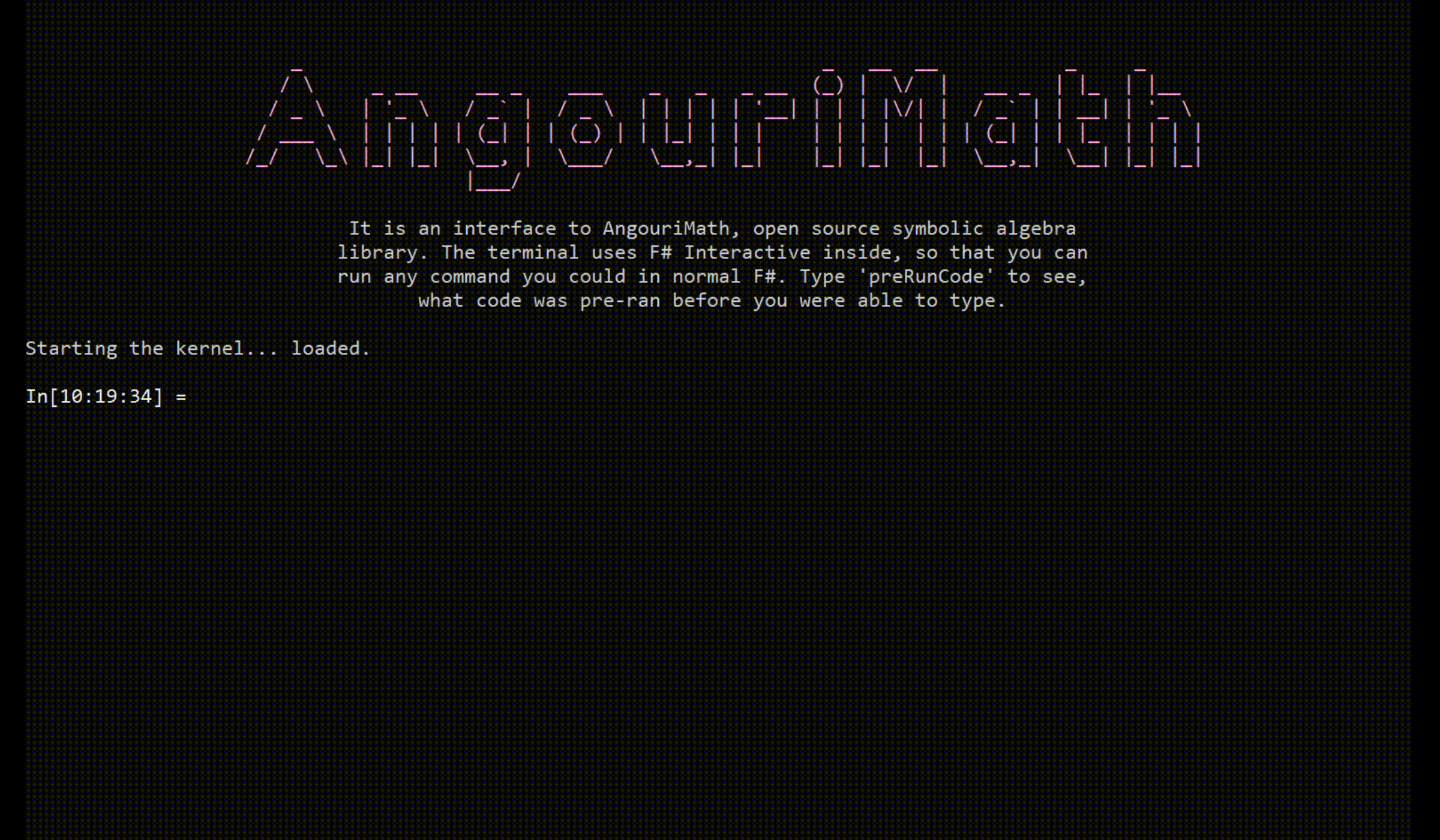By default, there is a number of opened modules, such as AggressiveOperators, which override the default arithmetic operators. To see what is ran beforehand, type preRunCode.

It can be easily used as an advanced calculator too, not only as some professional tool.

Angouri © 2019-2023 · Project's repo · Site's repo · Octicons · Transparency · 1534 pages online